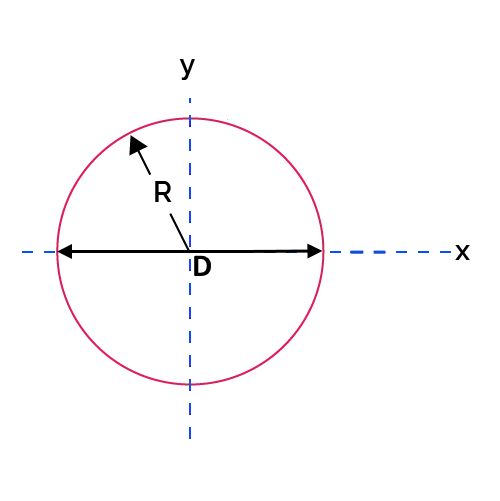# Polar Moment of Area Calculator

Created by Luis Hoyos
Last updated: Feb 12, 2023

With this tool, you can calculate the polar moment of area (also known as polar moment of inertia) of beams of solid and hollow circular shape.

The polar moment of inertia of circles is important, as circular elements are present in many mechanical engineering applications, as we'll explain in the rest of this article. For that reason, we created this calculator.

## Why do we need to calculate the polar moment of area?

Many machine elements in mechanical engineering are circular and experience torsional loads. Typical examples are power transmission shafts and bolts. Here's where the polar moment of inertia of a circle comes in. The polar moment is a geometrical property necessary to calculate the stress and deformation in those elements. It indicates their resistance to torsional loads.

For example, suppose you're designing a bolt element. In that case, you can:

1. Use our bolt torque calculator to forecast the necessary torque ($T$);
2. Calculate the polar moment of area ($J$) with this calculator; and
3. Once you know $T$ and $J$, you can use the maximum shear stress formula for torsion: $\tau_\text{max} = TR/J$, where $R$ is the beam radius (in this case, half the minor diameter of the bolt). PD: You can also calculate $\tau_\text{max}$ with our shear stress calculator.

Don't forget that, in the analysis of bolts, along with the shear stress, you must consider the axial stress (use our stress calculator) and the compressive forces that cause buckling (use our critical load calculator for that).

## Polar moment of inertia formulas

For a solid circular shaft or beam, we use the following formula for the polar moment of inertia (in terms of radius or diameter):

$J = \frac{\pi}{2}R^4 = \frac{\pi}{32}D^4$

, where:

• $J$ — Polar moment of inertia of the beam or shaft;
• $R$ — Shaft radius; and
• $D$ — Shaft diameter;

In a hollow circular section, we calculate the polar moment of area with the following equation:

$J = \frac{\pi}{2}(R^4 - {r}^4) \!=\! \frac{\pi}{32}(D^4 - {d}^4)$

, where:

• $r$ — Inner radius of the section; and
• $d$ — Inner diameter of the section;

Remember that the previous formulas apply to the polar moment of inertia of cylinder-like elements, like shafts or bolts.

Luis Hoyos
Select shape
Solid circular sectionin
or
Outer diameter (D)
in
Polar moment of inertia (J)
in⁴
People also viewed…

### Mohr's circle

Obtain the principal stresses from a plane stress state using our Mohr's circle calculator.